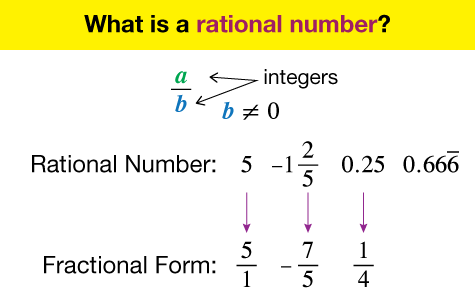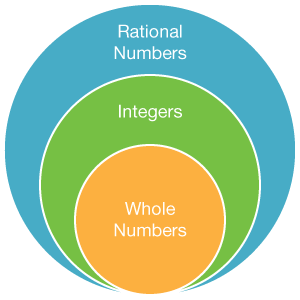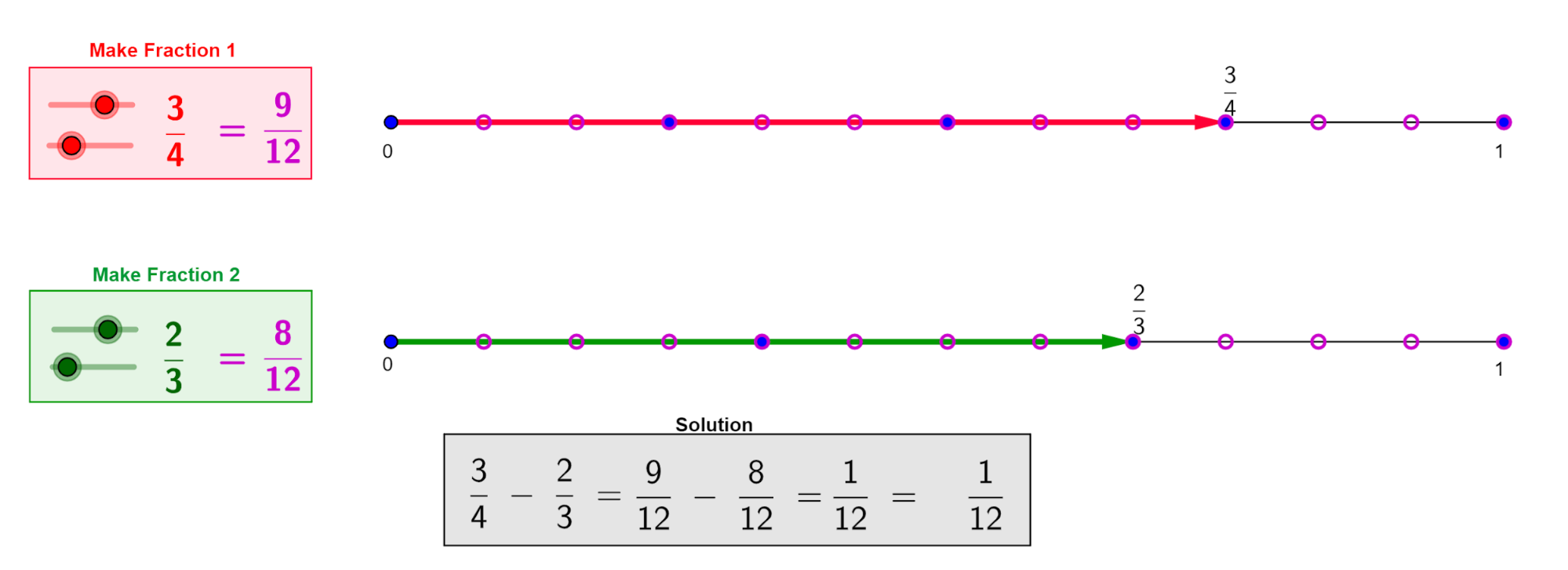# Operations on Rational Numbers

Go back to  'Rational Numbers'

## Introduction to Operations on Rational Numbers

I am sure you know that the number 5 can be also written as $${15 \over 3}$$? Similarly, the number $$3$$ can be written as $${3 \over 1}$$. When a number can be represented as a ratio of two numbers, then it is called a rational number. But there are certain criteria that these numbers should fulfil. First, the denominator should not be equal to zero. Next, the ratio should not be non-terminating. We spoke earlier of a standard ratio $$π$$ which is equal to $${22 \over 7}$$. and that is one example of a non-terminating decimal. So $$π$$ is not a rational number. We will be learning about rational numbers today, and also seeing how to carry out mathematical operations on them.## The Big Idea: Operations on Rational Numbers

### A simple idea: How do you operate using rational numbers?

We have learnt about fractions earlier, and we saw how different operators can be used on different fractions. Well, all the rules and principles that govern fractions can also be applied to rational numbers. The one thing to be kept in mind is that rational numbers also include negatives. So, while $${1 \over 5}$$ is a rational number, it is also true that $$-{1 \over 5}$$ is also a rational number.To understand the concept of negative rational numbers, we need to understand a number line. A number line is simply a line on which numbers are marked at equal intervals. A number line can be extended infinitely in both directions. One of the points of a number line is zero. All points to the right of the zero mark are positive numbers, while all the numbers to the left of zero are negative numbers.A number line also makes it very easy to visualize additions and subtractions of positive numbers and negative numbers. For example, if we wish to add $$-3$$ with $$+2,$$ then it means that the first number is three spaces to the left of zero, while the second number is two spaces to the right of zero. Therefore, their sum will be just one space to the left.

As we saw above, a rational number is a ratio of two numbers p and q, where q is non-zero number. Here p is called the numerator and q is called the denominator. When it comes to addition of two such rational numbers, there can be four possible variations.

First, both the rational numbers could have the same denominator. For example, when we wish to add $${3 \over 5}$$ and $${1 \over 5}$$$$,$$ the answer is simply the sum of 3 and 1, divided by the common denominator 5. So

\begin {align}\frac15+\frac35=\frac{(1+3)}5=\frac45\end {align}

Next, the two rational numbers could have the same denominator, but one of them could be negative. So, when you need to add $${3 \over 5}$$ and $$-{1 \over 5}$$$$,$$ then we can write the calculation in this way

\begin{align}\frac{3}{5} + \left( {- \frac{1}{5}} \right) &= \frac{{(3 + ( - 1))}}{5} \\&= \frac{{(3 - 1)}}{5} = \frac{2}{5}\end{align}

The third variant is when the two rational numbers to be added have different coefficients. Like we have seen earlier, we will make the two numbers similar to each other by taking the lowest common multiple of both denominators as the denominator of the answer. So, to add $${5 \over 6}$$ and $${7 \over 9}$$$$,$$ we first need to find the LCM of 6 and 9, which is 18. So, we can write $${5 \over 6}$$ as $${15 \over 18}$$ and $${7 \over 9}$$ as $${14 \over 18}$$$$.$$ Then the addition of these two rational numbers can be expressed in the following way

\begin {align}\frac56+\frac79=\frac{15\;}{18}+\frac{14}{18}=\frac{(15+14)}{18}=\frac{29}{18}\end {align}

The final variant is when one of the two rational numbers with different denominators is negative. So, if we need to add $${5 \over 6}$$ and $$-{7 \over 9}$$$$,$$ then the addition can be carried out in the following manner

\begin{align}\frac{5}{6} + \left( { - \frac{7}{9}} \right) &= \frac{{15\;}}{{18}} + \left( { - \frac{{14}}{{18}}} \right) \\&= \frac{{(15 + ( - 14))}}{{18}} \\&= \frac{1}{{18}}\end{align}

### Subtraction of rational numbers

If you can understand the concept of additive inverse, then you do not need to understand anything extra outside the addition we saw above, when we need to subtract two rational numbers. The additive inverse of a fraction is the number which when added to it gives a result zero. So, if you have a variable $$x,$$ and its additive inverse is $$i,$$ then $$x + i = 0, =\,> i = -x.$$ So, when expressed simply, the additive inverse of any number is the same number with a negative sign.

Now let us see how we can express how to subtract $${3 \over 7}$$ from $${5 \over 7}$$. The additive inverse of $${3 \over 7}$$ is $$-{3 \over 7}$$. So, the subtraction can be expressed as the addition to additive inverse.

Therefore,

\begin{align}\frac{5}{7} - \frac{3}{7} = \frac{5}{7} + \left( { - \frac{3}{7}} \right) = \frac{2}{7}\end{align}### Multiplication and Division of rational numbers

Just like we saw above that subtraction can be quite easily understood once addition is clear, similarly, division of two rational numbers is quite easy to comprehend once multiplication is clear. First, let us look at multiplication. When two rational numbers are to be multiplied together, then the simple thing to do is to multiply both numerators together to get the new numerator, and then the two denominators to get the new denominator. So when we multiply $${3 \over 5}$$ and $${4 \over 7}$$, the answer is \begin {align}\frac35\times\frac47=\frac{(3\times4)}{(5\times7)}=\frac{12}{35}\end {align}.

For division, we need to find the multiplicative inverse of the second rational number. Therefore \begin{align}\frac{{\left( {\frac{3}{4}} \right)}}{{\left( {\frac{5}{7}} \right)}} = \frac{3}{4} \times \frac{7}{5} = \frac{{(3 \times 7)}}{{(4 \times 5)}} = \frac{{21}}{{20}}\end{align}.

An Introduction to Rational Numbers
An Introduction to Rational Numbers